To Be “Continued” — an Unusual Fraction

Here is an interesting fraction to ponder: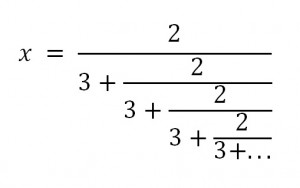Constructions such as these are called “continued fractions”. Though they go on forever, there is a way to evaluate them.  Before we do that, let’s get a feel for the answer we seek by figuring out an upper and lower limit on the value.

1.  x is less than 2/3.

We know this because the numerator is 2 and the denominator is 3 + something positive.  If the denominator is MORE than 3, then the fraction is LESS than 2/3.  But how much less?  Well, there is a limit on that as well…

2. x is more than 6/11.

As we just said, the denominator is more than 3, but how much more?  Look again at the “something positive” which is added to the denominator:  it is in fact the same expression x that we are hoping to find.  We don’t know its value, but we know it is less than 2/3.  So now we have: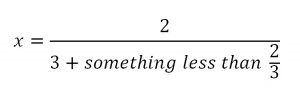The fraction will have its smallest value when the denominator is the biggest it can be.

That gives us a lower limit on the value of x: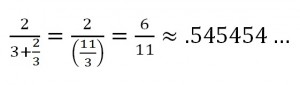But what if we want an exact value?  It turns out that we’ve already noticed the key to this puzzle.

Look again at the continued fraction.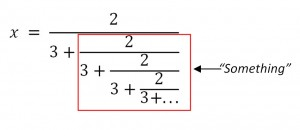The denominator is more than 3…but notice just how much more:  the expression added to 3 is the same as the original expression, x.

That means we can re-write the continued fraction this way:We multiply both sides by 3+x and distribute on the left hand side to get: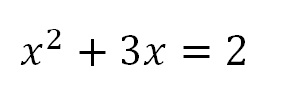Were you expecting that?  To evaluate the continued fraction, we have to solve a quadratic equation.  This means that we are going to have to choose a method.  I’m going to vote for completing the square (which I will return to in my next post).  But if you prefer, you can use the quadratic formula.  Either way, you’ll get: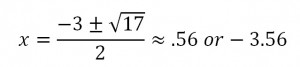It’s  the positive answer that we want here.  And its nice to see that it falls in the range we expected.

I still like completing the square better.  Before we get to that, here’s one more fun example that will end with a twist.

THE GOLDEN CONTINUED FRACTION: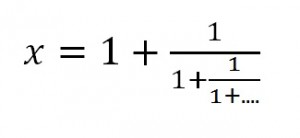Let’s call it an exercise (or two):

Show that x must be less than 2 and more than 1.5.

(You can post your reasoning in a comment if you like.)

But again, what if we want the exact value?  We have to notice that once again, the expression we seek is hidden inside the continued fraction. Here it is again: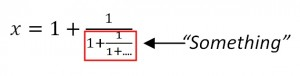Look closely – that “something” is the same expression we started with.  It’s x!

So we can re-write the original equation:And if we multiply both sides by x:Or: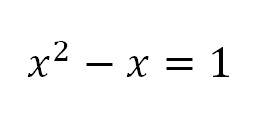We still have to solve this quadratic equation.  But those (both?) of you who have been following along with me might remember that we have seen this equation before.  This was exactly the quadratic equation we landed on when looking for the Golden Ratio.  The one equation answers two seemingly unrelated questions:

1. What is the value of this expression with a continued fraction?2. What is the aspect ratio of a rectangle from which you can remove a square, leaving behind a smaller rectangle with the same aspect ratio?

We’ll know both of these when we solve that quadratic equation.

(That was my idea of a cliff-hanger ending.)